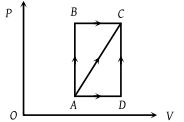A thermodynamic process is shown in the figure. The pressures and volumes corresponding to some points in the figure are : ${P}_{A}=3×{10}^{4}Pa,\text{\hspace{0.17em}}{P}_{B}=8×{10}^{4}Pa$ and ${V}_{A}=2×{10}^{-3}{m}^{3},\text{\hspace{0.17em}}{V}_{D}=5×{10}^{-3}{m}^{3}$. In process AB, 600 J of heat is added to the system and in process BC, 200 J of heat is added to the system. The change in internal energy of the system in process AC would be(1) 560 J

(2) 800 J

(3) 600 J

(4) 640 J

Concept Videos :-

#16 | Internal Energy (U) of Gas
#17 | Solved Example: 5
#18 | Solved Example: 6
#31 | First Law
#32 | Application of First Law to Adiabatic Process
#33 | Application of First Law to IsoThermal Process
#34 | Solved Example: 14
#35 | Application of First Law to Isochoric & Cyclic Processes
#36 | Solved Example: 15
#37 | Differential Form of First Law

Concept Questions :-

First law of thermodynamics